# Quotient

Determine the quotient and the second member of the geometric progression where a3=10, a1+a2=-1,6 a1-a2=2,4.

q =  -5
a2 =  -2

### Step-by-step explanation:

${a}_{2}=\left(-2\right)=-2$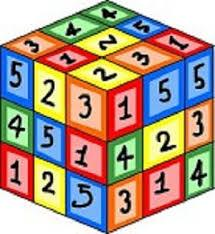Did you find an error or inaccuracy? Feel free to write us. Thank you!## Related math problems and questions:

• Intersect and conjuctionLet U={1,2,3,4,5,6} A={1,3,5} B={2,4,6} C={3,6} Find the following. 1. )AUB 2. )A'UB'
• Evaluation of expressionsIf a2-3a+1=0, find (i)a2+1/a2 (ii) a3+1/a3
• Five element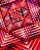The geometric sequence is given by quotient q = 1/2 and the sum of the first six members S6 = 63. Find the fifth element a5.
• Expressions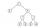Find out value of expressions if a = -1, b =2: x=b - 2a - ab y=a3 - b2 - 2ab z=a2 b3 - a3 b2 w=a + b + a3 - b2
• GP membersThe geometric sequence has 10 members. The last two members are 2 and -1. Which member is -1/16?
• CoefficientDetermine the coefficient of this sequence: 7.2; 2.4; 0.8
• Tenth memberCalculate the tenth member of geometric sequence when given: a1=1/2 and q=2
• Solve equationsolve equation: [(a2)+3]1/2 + [(a2)-3]1/2 = 5
• Geometric mean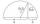Calculate the geometric mean of numbers a=15.2 and b=25.6. Determine the mean by construction where a and b are the length of the lines.
• Geometric progression 2There is geometric sequence with a1=5.7 and quotient q=-2.5. Calculate a17.
• HP - harmonic progressionDetermine the 8th term of the harmonic progression 2, 4/3, 1,…
• Fifth memberDetermine the fifth member of the arithmetic progression, if the sum of the second and fifth members equal to 73, and difference d = 7.
• Sequence 11What is the nth term of this sequence 1, 1/2, 1/3, 1/4, 1/5?
• Geometric seqFind the third member of geometric progression if a1 + a2 = 36 and a1 + a3 = 90. Calculate its quotient.
• If the 3If the 6th term of a GP is 4 and the 10th is 4/81, find common ratio r.
• Q of GP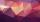Calculate quotient of geometric progression if a1=5 a a1+a2=12.
• Gp - 80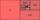One of the first four members of a geometric progression is 80. Find its if we know that the fourth member is nine times greater than the second.